Latest SSC jobs   »   Reasoning Quiz For SSC CHSL :...

# Reasoning Quiz For SSC CHSL : 12th February 2020 For Series , Syllogism

Direction (1-3): A series is given with one term /number missing. Choose the correct alternative from the given ones that will complete the series.

Q1. PRQ, TVU, YAZ, ?
(a) BDC
(b) EGF
(c) DFE
(d) EFG

Q2. 283, 94, 31, 10, ?
(a) 3
(b) 4
(c) 5
(d) 7

Q3. In the following question, two statements are given each followed by two conclusions I and II. You have to consider the statements to be true even if they seem to be at variance from commonly known facts. You have to decide which of the given conclusions, if any, follows from the given statements.
Statements: (I) Some bags are hot.
(II) All hot things are cakes.
Conclusion: (I) All cakes are bags.
(II) All bags are cakes.

(a) Conclusion I follows
(b) Conclusion II follows
(c) Neither I nor II follows
(d) Both I and II follows

Direction (4-5): In each of the following question below are given some statements followed by some conclusions. Taking the given statements to be true even if they seem to be at variance from commonly known facts, read all the conclusions and then decide which of the given conclusion logically follows the given statements.

Q4. Statements:
I. All big are small.
II. Some small are tall.
III. No tall is white.
Conclusions:
I. Some big are white.
II. Some big are not white.
III. Some small are not white.

(a) Only conclusion (I) and (II) follow
(b) Only conclusion (II) and (III) follow
(c) conclusion (III) follows and either (I) OR (II)
(d) None conclusion follows

Q5 Statements:
I. Some cup are hot.
II. All hot are tea.
Conclusions:
I. Some tea are cup.
II. All cups are tea.

(a) Only conclusion (I) follows
(b) Only conclusion (II) follows
(c) Both conclusions follow
(d) Neither conclusion (I) nor conclusion (II) follows

Q6. There are two statements labeled as Assertion (A) and Reason (R).
Assertion (A): Dyslexia is a psychological disorder.
Reason (R): Vitamin deficiency causes the disease.
Choose the correct option.
Codes:
(a) If both (A) and (R) are ture.
(b) If both (A) and (R) are false.
(c) If (A) is false, but (R) is true.
(d) If (A) is true, but (R) is false.

Directions (7-8): In the following questions, two statements are given followed by four/two conclusions I, II, III & IV. You have to consider two statements to be true even if they seem to be at variance from commonly known facts. You have to decide which of the given conclusions, if any, follow from the given statements.

Q7. Statements
(A) All books are trees.
(B) All trees are lions.
Conclusions
I. All books are lions.
II. All lions are books.
III. All trees are books.
IV. Some lions are books.

(a) Only II and III follow.
(b) Only I and IV follow.
(c) None of the conclusions follows.
(d) All conclusions follow.

Q8. Statements
(A) All men are employed.
(B) No employees are professionals.
Conclusions
I. No men are employed.
II. No men are professionals.

(a) Only I follows.
(b) Only II follows.
(c) Neither I nor II follows.
(d) Both I and II follow.

Direction (9-10); In each of the following question below are given some statements followed by some conclusions. Taking the given statements to be true even if theyseem to be at variance from commonly known facts, read all the conclusions and then decide which of the given conclusion logically follows the given statements.

Q9. Statements:
I. Some knives are spoons.
II. Some forks are knives.
III. No fork is a green.
Conclusions:
I. Some spoons are forks.
II. Some knives are green.
III. Some fork are not knives.
IV. Some knives are not green.
(a) Only conclusion (IV) follows
(b) Only conclusion (III) follows
(c) Only conclusion (III) and (I) follow
(d) Only conclusion (I) and (II) follow

Q10 Statements:
I. Some mangoes are not red.
II. All red are raw.
III. Some raw are mangoes.
Conclusions:
I. Some mangoes are not raw.
II. Some red are not mangoes.
III. All raw are red.
(a) Only conclusion (I) follows
(b) Only conclusion (II) and (III) follow
(c) Only conclusion (I) and (III) follow
(d) No conclusion follows

Solutions: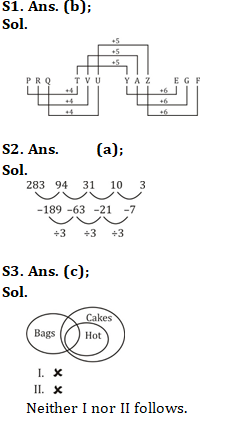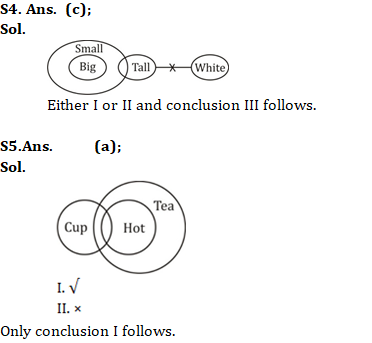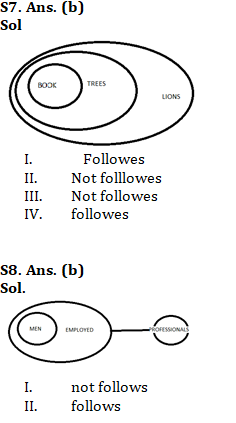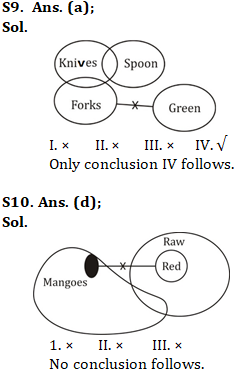You may also like to read:

#### Congratulations!General Awareness & Science Capsule PDFIncorrect details? Fill the form again here

General Awareness & Science Capsule PDF

Thank You, Your details have been submitted we will get back to you.
•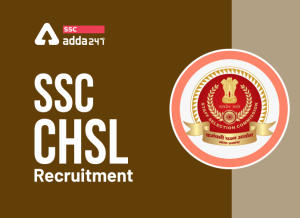SSC CHSL 2022 Notification, Online Appli...
•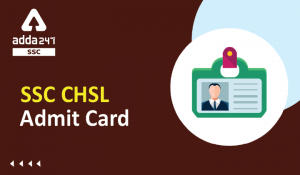SSC CHSL Admit Card 2022, Download SSC C...
•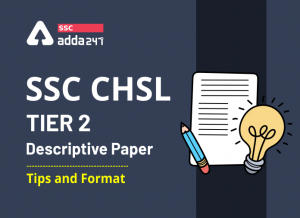SSC CHSL Tier 2 Descriptive Paper | Tips...
•SSC CHSL Salary: Check In Hand Salary Af...
•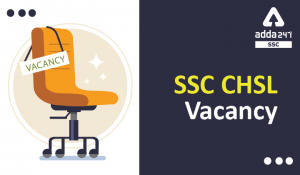SSC CHSL 2022 Vacancy Released, Check Po...
•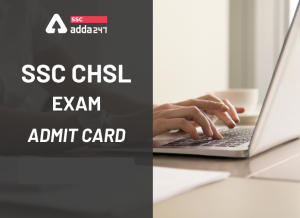SSC CHSL Skill Test Admit Card 2022, Dow...